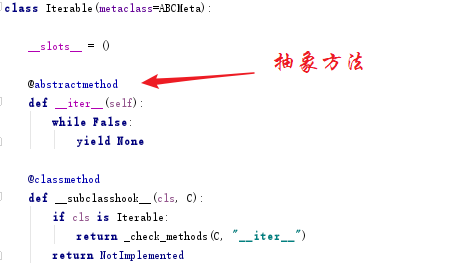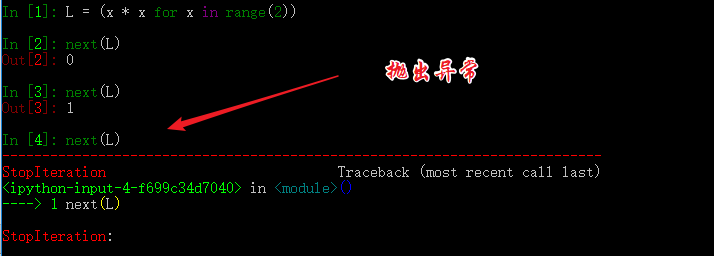# Python并发编程之从生成器使用入门协程（七）

## 本文目录

• 可迭代、迭代器、生成器
• 如何运行/激活生成器
• 生成器的执行状态
• 生成器的异常处理
• 从生成器过渡到协程：yield

### . 可迭代、迭代器、生成器

import collectionsfrom collections.abc import Iterable, Iterator, Generator# 字符串astr = "XiaoMing"print("字符串：{}".format(astr))print(isinstance(astr, Iterable))print(isinstance(astr, Iterator))print(isinstance(astr, Generator))# 列表alist = [21, 23, 32,19]print("列表：{}".format(alist))print(isinstance(alist, Iterable))print(isinstance(alist, Iterator))print(isinstance(alist, Generator))# 字典adict = {"name": "小明", "gender": "男", "age": 18}print("字典：{}".format(adict))print(isinstance(adict, Iterable))print(isinstance(adict, Iterator))print(isinstance(adict, Generator))# dequeadeque=collections.deque('abcdefg')print("deque：{}".format(adeque))print(isinstance(adeque, Iterable))print(isinstance(adeque, Iterator))print(isinstance(adeque, Generator))

字符串：XiaoMingTrueFalseFalse列表：[21, 23, 32, 19]TrueFalseFalse字典：{'name': '小明', 'gender': '男', 'age': 18}TrueFalseFalsedeque：deque(['a', 'b', 'c', 'd', 'e', 'f', 'g'])TrueFalseFalsefrom collections.abc import Iterable, Iterator, Generatorclass MyList(object):  # 定义可迭代对象类    def __init__(self, num):        self.end = num  # 上边界    # 返回一个实现了__iter__和__next__的迭代器类的实例    def __iter__(self):        return MyListIterator(self.end)class MyListIterator(object):  # 定义迭代器类    def __init__(self, end):        self.data = end  # 上边界        self.start = 0    # 返回该对象的迭代器类的实例；因为自己就是迭代器，所以返回self    def __iter__(self):        return self    # 迭代器类必须实现的方法，若是Python2则是next()函数    def __next__(self):        while self.start < self.data:            self.start += 1            return self.start - 1        raise StopIterationif __name__ == '__main__':    my_list = MyList(5)  # 得到一个可迭代对象    print(isinstance(my_list, Iterable))  # True    print(isinstance(my_list, Iterator))  # False    # 迭代    for i in my_list:        print(i)    my_iterator = iter(my_list)  # 得到一个迭代器    print(isinstance(my_iterator, Iterable))  # True    print(isinstance(my_iterator, Iterator))  # True    # 迭代    print(next(my_iterator))    print(next(my_iterator))    print(next(my_iterator))    print(next(my_iterator))    print(next(my_iterator))

01234TrueFalseTrueTrue01234

from collections.abc import IteratoraStr = 'abcd'  # 创建字符串，它是可迭代对象aIterator = iter(aStr)  # 通过iter()，将可迭代对象转换为一个迭代器print(isinstance(aIterator, Iterator))  # Truenext(aIterator)  # anext(aIterator)  # bnext(aIterator)  # cnext(aIterator)  # d

yield 是什么东西呢，它相当于我们函数里的return。在每次next()，或者for遍历的时候，都会yield这里将新的值返回回去，并在这里阻塞，等待下一次的调用。正是由于这个机制，才使用生成器在Python编程中大放异彩。实现节省内存，实现异步编程。

• 使用列表生成式
# 使用列表生成式，注意不是[]，而是()L = (x * x for x in range(10))print(isinstance(L, Generator))  # True
• 实现yield的函数
# 实现了yield的函数def mygen(n):    now = 0    while now < n:        yield now        now += 1if __name__ == '__main__':    gen = mygen(10)    print(isinstance(gen, Generator))  # True

### . 如何运行/激活生成器

• 使用next()
• 使用generator.send(None)

def mygen(n):    now = 0    while now < n:        yield now        now += 1if __name__ == '__main__':    gen = mygen(4)    # 通过交替执行，来说明这两种方法是等价的。    print(gen.send(None))    print(next(gen))    print(gen.send(None))    print(next(gen))

0123

### . 生成器的执行状态

GEN_CREATED # 等待开始执行
GEN_RUNNING # 解释器正在执行（只有在多线程应用中才能看到这个状态）
GEN_SUSPENDED # 在yield表达式处暂停
GEN_CLOSED # 执行结束

from inspect import getgeneratorstatedef mygen(n):    now = 0    while now < n:        yield now        now += 1if __name__ == '__main__':    gen = mygen(2)    print(getgeneratorstate(gen))    print(next(gen))    print(getgeneratorstate(gen))    print(next(gen))    gen.close()  # 手动关闭/结束生成器    print(getgeneratorstate(gen))

GEN_CREATED0GEN_SUSPENDED1GEN_CLOSED

### . 生成器的异常处理def mygen(n):    now = 0    while now < n:        yield now        now += 1    raise StopIterationif __name__ == '__main__':    gen = mygen(2)    next(gen)    next(gen)    next(gen)

### . 从生成器过渡到协程：yield

def jumping_range(N):    index = 0    while index < N:        # 通过send()发送的信息将赋值给jump        jump = yield index        if jump is None:            jump = 1        index += jumpif __name__ == '__main__':    itr = jumping_range(5)    print(next(itr))    print(itr.send(2))    print(next(itr))    print(itr.send(-1))

0232

• yield index 是将index return给外部调用程序。
• jump = yield 可以接收外部程序通过send()发送的信息，并赋值给jumpposted @ 2018-05-19 17:21  王一白  阅读(6852)  评论(4编辑  收藏  举报